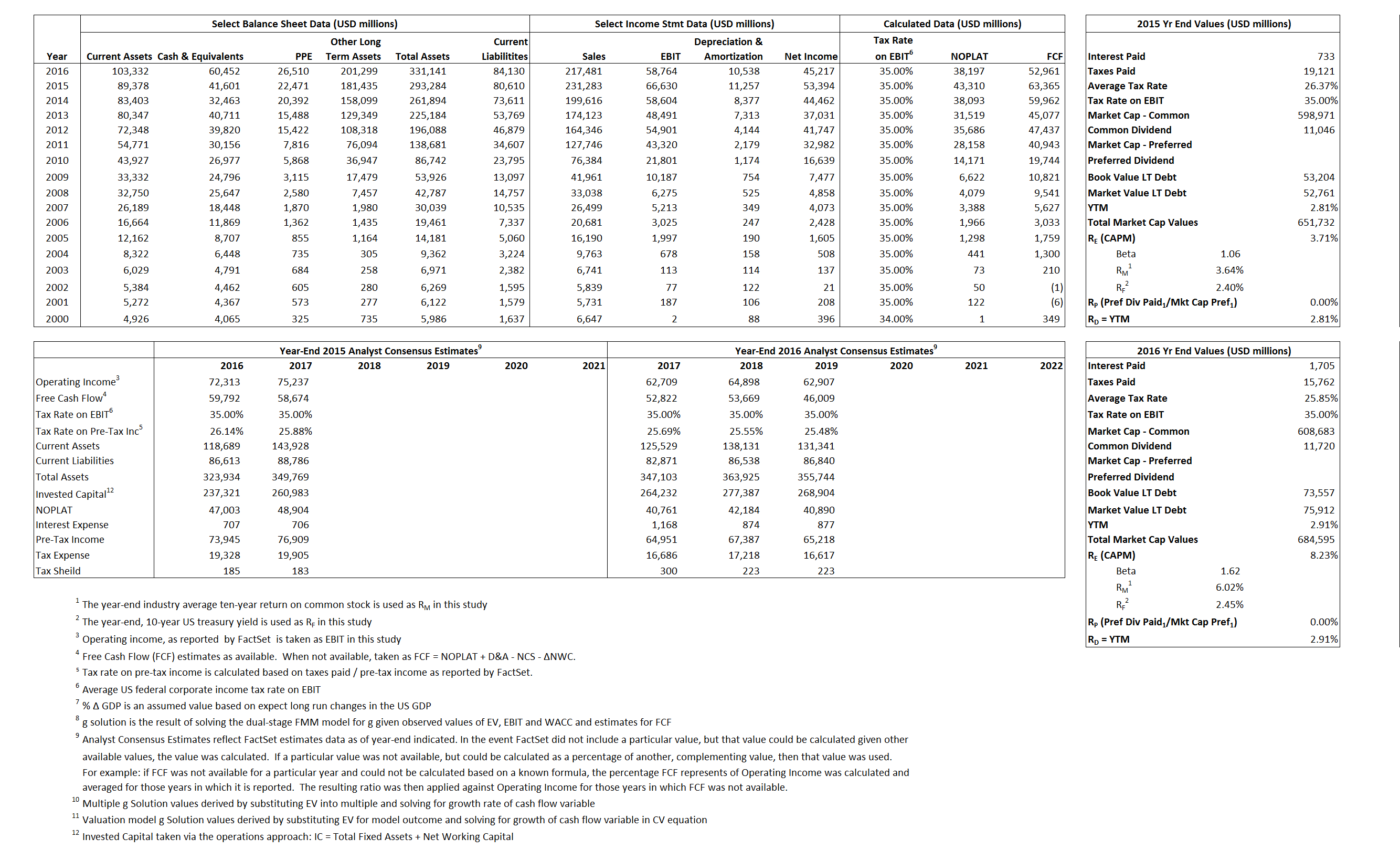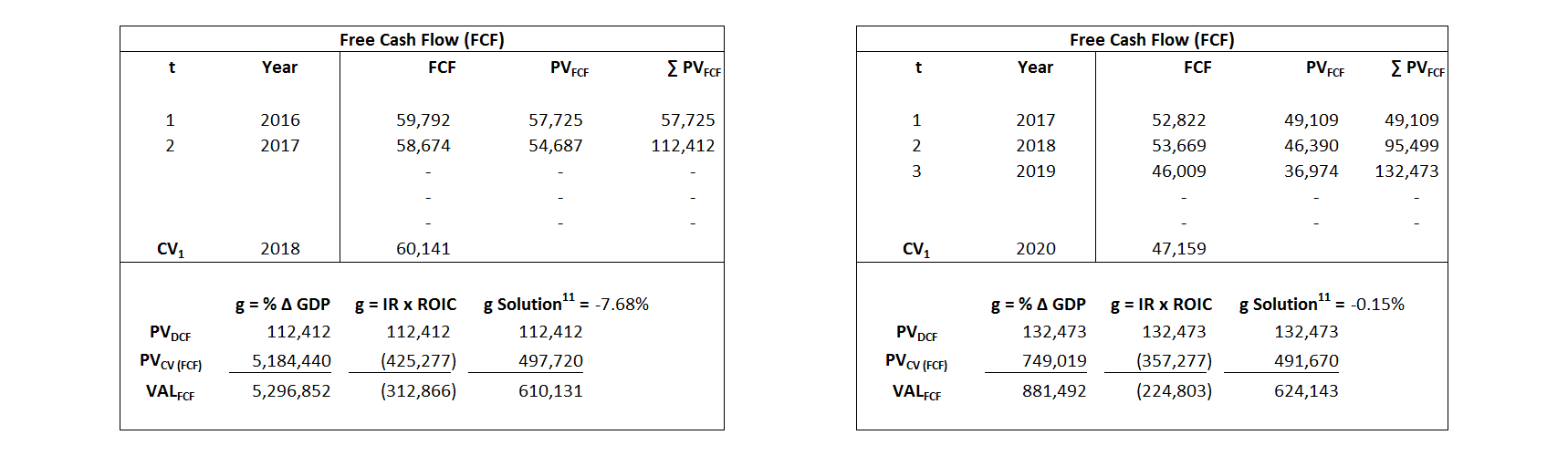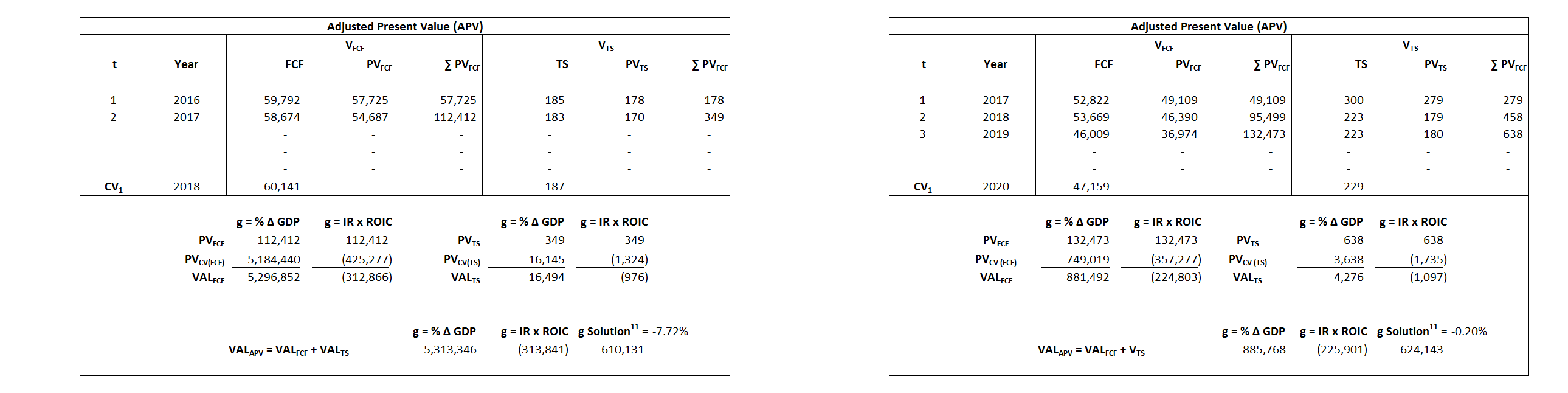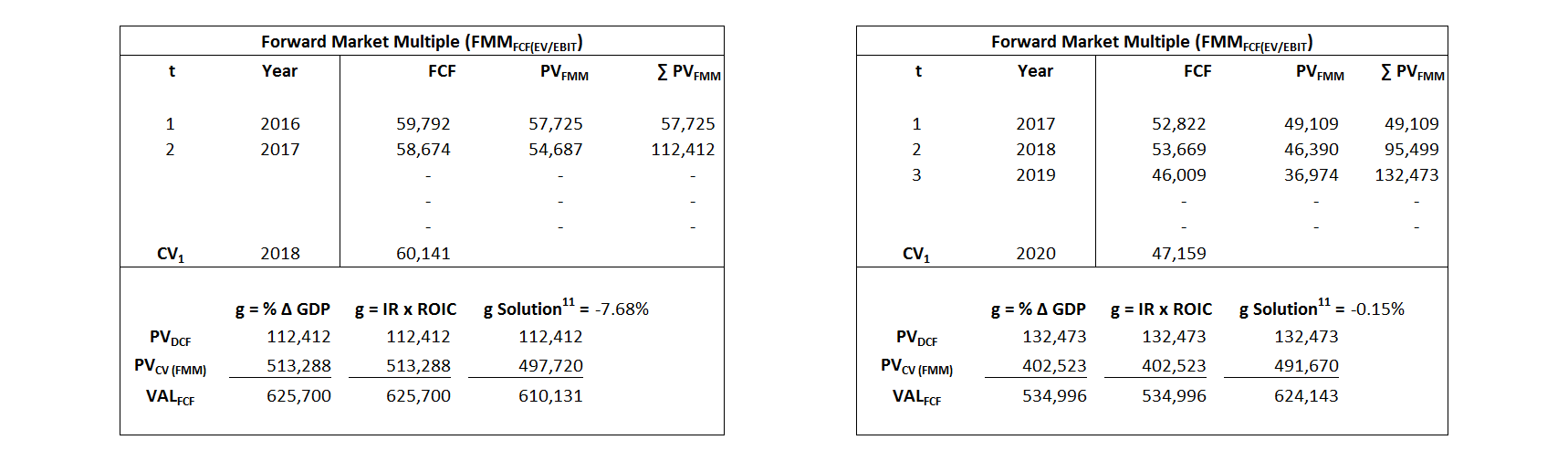# Apple

## Analyst Listing

The following analysts provide coverage for the subject firm as of May 2016:

 Broker Analyst Analyst Email Stifel Nicolaus Aaron Rakers rakersa@stifel.com Mizuho Securities USA Abhey Lamba abhey.lamba@us.mizuho-sc.com RBC Capital Markets Amit Daryanani amit.daryanani@rbccm.com Brean Capital, LLC Ananda Baruah abaruah@breancapital.com Oppenheimer Andrew Uerkwitz andrew.uerkwitz@opco.com Pacific Crest Securities-KBCM Andy Hargreaves ahargreaves@pacific-crest.com Pacific Crest Andy Hargreaves ahargreaves@pacific-crest.com William Blair Anil Doradla adoradla@williamblair.com Drexel Hamilton Brian J. White bwhite@drexelhamilton.com BGC Financial, L.P. Colin W. Gillis cgillis@bgcpartners.com Piper Jaffray Gene Munster gene.a.munster@pjc.com Atlantic Equities James Cordwell j.cordwell@atlantic-equities.com Nomura Research Jeffrey Kvaal jeffrey.kvaal@nomura.com Rosenblatt Securities Jun Zhang jzhang@rblt.com Credit Suisse Kulbinder Garcha kulbinder.garcha@credit-suisse.com Needham Laura Martin lmartin@needhamco.com Wells Fargo Securities Maynard J. Um maynard.um@wellsfargo.com Maxim Group Nehal Chokshi maynard.um@wellsfargo.com CRT Capital Group Rob Cihra rcihra@sterneageecrt.com Cross Research Shannon Cross shannon@crossresearch.com FBN Securities Shebly Seyrafi sseyrafi@fbnsecurities.com Deutsche Bank Research Sherri Scribner sherri.scribner@db.com Hilliard Lyons Stephen Turner sturner@hilliard.com Canaccord Genuity T. Michael Walkley mwalkley@canaccordgenuity.com Raymond James Tavis C. McCourt tavis.mccourt@raymondjames.com BMO Capital Markets Tim Long tim.long@bmo.com Cowen & Company Timothy Arcuri timothy.arcuri@cowen.com Bernstein Research Toni M. Sacconaghi Jr sacconaghi@bernstein.com BTIG Walter Piecyk wpiecyk@btig.com Daiwa Securities Co. Ltd. Yoko Yamada yoko.yamada@us.daiwacm.com

## Primary Input Data## Derived Input Data

### Equational Form

Net Operating Profit Less Adjusted Taxes NOPLAT 43,310  38,197$NOPLAT\, =\, EBIT\, x\, (1 \,-\, Avg \,\,Tax\,\, Rate\,\, on\,\, EBIT)$
Free Cash Flow FCF  63,3645   52,961$FCF\,=NOPLAT\,+\,Non-Cash\,Expenses-\Delta NWC\,-\,NCS$
Tax Shield TS  193  441$TS\,=\,Interest\,\,Paid\,\,x\,\, Avg \,\,Tax\,\,Rate\,\, on\,\, Pre-Tax\,\, Income$
Invested Capital IC  212,674  247,011$IC\,=\,Fixed\,\,Operating\,\,Assets\,\,+\,\,Net\,\, Working\,\, Capital$
Return on Invested Capital ROIC  20.36% 15.46%$ROIC\,=\,\frac { NOPLAT }{ IC }$
Net Investment NetInv   35,648  44,875$NetInv\,=\,{ {IC}_{1}}-{{IC}_{0}}+Depreciation$
Investment Rate IR  82.31% 117.48%$IR\,=\,\frac {NetInv}{NOPLAT}$
Weighted Average Cost of Capital WACCMarket 3.58% 7.56%$WACC\,=\,\frac { E }{ V } { R }_{ E }\,+\,\frac { P }{ V } { R }_{ P }\,+\,\frac { D }{ V } { R }_{ D }\left( 1- Avg\,\, Tax\,\,Rate\,\,on\,\,Pre-Tax\,\,Income \right)$
WACCBook  9.44% 9.15%
Enterprise value EVMarket  610,131  624,143$EV\,=\,Market\,\,Cap\,\,Equity\,+\,\,Long\,\,Term\,\,Debt\,-\,Cash$
EVBook  610,574  621,788
EV/EBIT Multiple$\frac{EV_{Market}}{EBIT}$  610,131  10.62$EV/EBIT\,=\,\frac { EV}{ EBIT}$
Long-Run Growth g = IR x ROIC
16.76%  18.17% Long-run growth rates of the income variable  are used in the Continuing Value portion of the valuation models.
g = %$\Delta$ GDP  2.50% 2.50%

## Valuation Model Outcomes

The outcomes presented in this study are the result of original input data, derived data, and synthesized inputs and, depending on the equational form of any particular valuation model, may result in irrelevant or implausible results.  For example, in the event WACC < g, the value of this term, often found in the denominator of an equation’s continuation value term, will be expressly negative and may result in a negative overall valuation for the firm.  In the event of a WACC < g relation, the model form as applied to the subject firm offers an irrelevant outcome.

### Equational form

Key Value Driver (NOPLAT) KVD (NOPLAT)${ Value }_{ DCF/KVD }=\sum { \frac { NOPLAT_{ t } }{ { \left( 1+WACC \right) }^{ t } } +\frac { \frac { { NOPLAT }_{ 1 }\left( 1-\frac { g }{ ROIC } \right) }{ WACC-g } }{ { \left( 1+WACC \right) }^{ t } } }$Key Value Driver (FCF) KVD (FCF)${ Value }_{ DCF/KVD }=\sum { \frac { FCF_{ t } }{ { \left( 1+WACC \right) }^{ t } } +\frac { \frac { { NOPLAT }_{ 1 }\left( 1-\frac { g }{ ROIC } \right) }{ WACC-g } }{ { \left( 1+WACC \right) }^{ t } } }$Free Cash Flow FCF${ Value }_{ DCF/FCF }=\sum { \frac { FCF_{ t } }{ { \left( 1+WACC \right) }^{ t } } +\frac { \frac { { FCF }_{ 1 }}{ WACC-g } }{ { \left( 1+WACC \right) }^{ t } } }$Economic Profit ECON π${ Value }_{ { ECON\pi } }= I{ C }_{ 0 }+\sum { \frac { { IC }_{ t-1 }(ROI{ C }_{t}-WAC{C}_{t}) }{ { \left( 1+WACC \right) }^{ t } }+ \frac {\frac { I{C}_{0}\ x\ (ROI{C}_{1}\ -\ WAC{C}_{1}) }{ WACC-g } }{ { \left( 1+WACC \right) }^{ t } } }$Adjusted Present Value APV${ Value }_{ APV }=\sum { \frac { FCF_{ t } }{ { \left( 1+{ k }_{ u } \right) }^{ t } } +\frac { \frac { { FCF }_{ 1 }}{ { k }_{ u }-g } }{ { \left( 1+{ k }_{ u } \right) }^{ t } } } +\sum { \frac { { TS }_{ t } }{ { \left( 1+{ k }_{ tax } \right) }^{ t } } +\frac { \frac { { TS }_{ 1 }}{ { k }_{ tax }-g } }{ { \left( 1+{ k }_{ tax } \right) }^{ t } } }$Forward Market Multiple FMM${ Value }_{ DCF/FMM}=\sum { \frac { FCF_{ t } }{ { \left( 1+WACC \right) }^{ t } } +\frac { { EBIT }_{ 1 }\,{x}\,{FMM}}{ { \left( 1+WACC \right) }^{ t } } }{\,\,\,; \,\,FMM\,=\,\frac{{EV}_{t=0}}{{EBIT}_{t=0}}}$